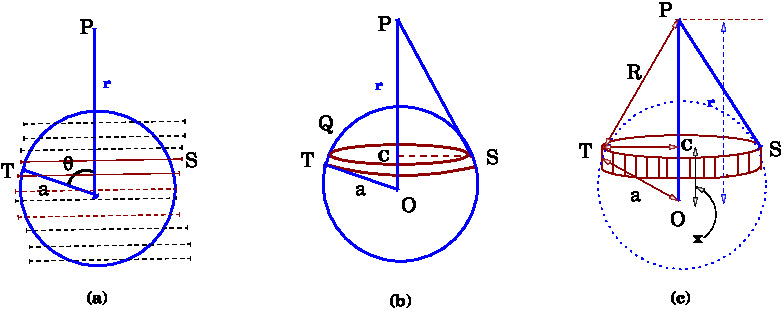# [YMP/EM-02007] Electric Field of a Uniformly Charged Spherical Shell

For page specific messages
For page author info

Category:

$\newcommand{\mHighLight}[]{#2}$
The surface charge density of a thin spherical shell is given to be uniform $\sigma$. We know that the field due to a ring for a point on the axis of the ring at a distance $$z$$ is
\begin{eqnarray}
\vec{E}_z&=&\frac{qz}{4\pi\epsilon_0(z^2+r_0^2)^{3/2}} \label{eq13}
\end{eqnarray}
Now, we need to compute the electric field due to a thin spherical shell at a point $$P$$.
The origin $$O$$ of coordinate system  is taken at the centre of the sphere. We divide the shell into thin strips of circular rings with planes perpendicular to the line $$OP$$ as shown in Fig (a) below.We consider a strip lying between $$x$$ and $$x+dx$$ as in Fig (b).
Then we have the expressions of  different lengths as follows, see (c).

• Distance between the center of the shell and the strip= radius of the shell =$$OT$$ = $$a$$.
• Distance of the center, $$O$$, of the shell from the field point $$P$$ = $$OP$$= $$r$$.
• Distance of the center of the shell, $$O$$, from the center, $$C$$, of the strip = $$OC$$  =$$x =a \cos\theta$$.
• The radius of the strip$$= CT = r_0 = a \sin \theta$$.

Consider a ring shaped strip, as above, lying between $$x$$ and $$x+dx$$. The corresponding range of angles $\theta$ and $\theta+d\theta$ is shown in the figure. This slice is a ring of radius $r_0$ and is at a distance $$x$$ from the centre.  Then
where $$a$$ denotes the radius of the shell and $$z$$ is the distance of the slice from the point $$P$$.

The ring can be regarded as a closed strip  of length $$2\pi r_0$$ and width $$ad\theta$$. Therefore, its area is $$dS=2\pi r_0 a d\theta$$ and the charge on the strip will be $dq = \sigma dS = \sigma (2\pi r_0 a d\theta)$

We will express the charge in terms of the radius $$a$$ and the total charge $$Q$$ on the sphere. Noting $$Q=\sigma (4\pi a^2)$$, we get
\begin{eqnarray}
\nonumber dq&=&(ad\theta)(2\pi a \sin \theta) \sigma
=\Big(\frac{Q}{4\pi a^2}\Big) a^2 (2\pi\sin \theta\, d\theta) \\
\text{or  } dq&=&\frac{Q}{2}\sin \theta\, d\theta \label{eq15}
\end{eqnarray}
Using the result for the field of a ring we get electric field of the ring as \begin{equation} dE = \frac{dq}{4\pi \epsilon_0}\frac{1}{R^2} \label{EQ22} \end{equation} where  is the distance $$PT$$ of the point $$P$$ from the ring. We need to express $$R$$ in terms of $$a$$ and $$\theta$$. From (c) we note that
\begin{eqnarray}
R^2 &=& CT^2+ CP^2\\
&=& r_0^2 + (r-x)^2\\
&=&a^2 \sin^2\theta + (r-a \cos\theta)^2\\
&=& r^2+a^2 -2ar \cos\theta.
\end{eqnarray}
Using this expression for $$R$$ in \eqref{EQ22} and integrating over $$\theta$$ we get\begin{eqnarray}
E&=&\frac{1}{4\pi\epsilon_0}\int_0^{\pi}\frac{Q\sin \theta
d\theta(r-a\cos\theta)}{2(r^2+a^2-2ar\cos\theta)^{3/2}}\\
\nonumber&=&\frac{Q}{4\pi\epsilon_0}\int_{-1}^{1}\frac{
\nonumber&=&\frac{1}{2}\frac{Q}{4\pi\epsilon_0}\int_{-1}^{1}\frac{1}{r}\frac{
r^2-atr}{(r^2+a^2-2art)^{3/2}}dt\\
\nonumber&=&\frac{1}{2r}\frac{Q}{4\pi\epsilon_0}\int_{-1}^{1}
\frac{\mHighLight[lime]{$r^2$}+\frac{1}{
2}(r^2+a^2-2atr)-\mHighLight[lime]{\frac{1}{2}(r^2+a^2)}}{(r^2+a^2-2art)^{3/2}}dt\\
\nonumber&=&\frac{1}{2r}\frac{Q}{4\pi\epsilon_0}\int_{-1}^{1}
\Big[\frac{1}{2}\frac{\mHighLight[lime]{
(r^2-a^2)}}{(r^2+a^2-2art)^{3/2}}dt+\frac{1}{2}
\frac{(dt)}{(r^2+a^2-2art)^{1/2}}\Big]\\
\nonumber&=&\frac{1}{2r}\frac{Q}{4\pi\epsilon_0}\Big[\frac{1}{2}\frac{(r^2-a^2)}{
ar(r^2+a^2-2art)^{1/2}} - \frac{1}{2ar}(r^2+a^2-2art)^{1/2}\Big]_{-1}^{+1}
\label{eq16}
\end{eqnarray} Before proceeding further, it is important to note that
$\sqrt{r^2+a^2-2ar}=|a-r|= \begin{cases} r-a, & \text{ if } r>a \\ a-r, & \text{ if } r<a. \end{cases}$
Case $r>a$:
\begin{eqnarray}
\nonumber{E}&=&\frac{1}{2ar}\frac{Q}{8\pi\epsilon_0r}\left[\left\{\frac{(r^2-a^2)}{r-a}
-  (r-a)\right\}- \left\{\frac{r^2-a^2}{r+a}  - (r+a)\right\}\right]\\
\nonumber&=&\frac{1}{2ar}\frac{Q}{8\pi\epsilon_0r}\big[\{(r+a) - (r-a)\}- \{(r-a)-
(r+a)\}\big]\\
&=&\frac{Q}{4\pi\epsilon_0r^2} \label{eq17}
\end{eqnarray}
Case $r<a$:
\begin{eqnarray}
\nonumber {E}&=&\frac{1}{2ar}\frac{Q}{8\pi\epsilon_0r}\Big[\Big\{\frac{(r^2-a^2)}{a-r}
- (a-r)\Big\}- \Big\{\frac{r^2-a^2}{r+a}  - (r+a)\Big\}\Big]\\
\nonumber&=&\frac{1}{2ar}\frac{Q}{8\pi\epsilon_0r}\big[\big\{-(r+a) - (a-r)\big\}- \big\{(r-a)-
(r+a)\big\}\big]\\
&=&0. \label{eq18}
\end{eqnarray}

n
0

X